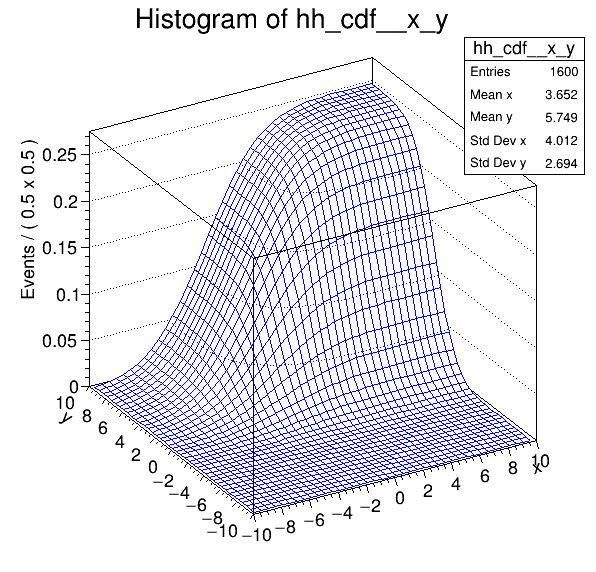Rf 3 0 8_Normintegration 2D¶

Multidimensional models: normalization and integration of pdfs, construction of cumulative distribution functions from pdfs in two dimensions

Author: Wouter Verkerke
This notebook tutorial was automatically generated with ROOTBOOK-izer from the macro found in the ROOT repository on Monday, January 17, 2022 at 09:59 AM.

In :
%%cpp -d
#include "RooRealVar.h"
#include "RooGaussian.h"
#include "RooConstVar.h"
#include "RooProdPdf.h"
#include "RooAbsReal.h"
#include "RooPlot.h"
#include "TCanvas.h"
#include "TAxis.h"
#include "TH1.h"
In :
%%cpp -d
// This is a workaround to make sure the namespace is used inside functions
using namespace RooFit;

Setup model¶

Create observables x,y

In :
RooRealVar x("x", "x", -10, 10);
RooRealVar y("y", "y", -10, 10);
RooFit v3.60 -- Developed by Wouter Verkerke and David Kirkby
Copyright (C) 2000-2013 NIKHEF, University of California & Stanford University

Create pdf gaussx(x,-2,3), gaussy(y,2,2)

In :
RooGaussian gx("gx", "gx", x, RooConst(-2), RooConst(3));
RooGaussian gy("gy", "gy", y, RooConst(+2), RooConst(2));

Create gxy = gx(x)*gy(y)

In :
RooProdPdf gxy("gxy", "gxy", RooArgSet(gx, gy));

Retrieve raw & normalized values of roofit p.d.f.s¶

Return 'raw' unnormalized value of gx

In :
cout << "gxy = " << gxy.getVal() << endl;
gxy = 0.485672

Return value of gxy normalized over x and y in range [-10,10]

In :
RooArgSet nset_xy(x, y);
cout << "gx_Norm[x,y] = " << gxy.getVal(&nset_xy) << endl;
gx_Norm[x,y] = 0.0129332

Create object representing integral over gx which is used to calculate gx_Norm[x,y] == gx / gx_Int[x,y]

In :
RooAbsReal *igxy = gxy.createIntegral(RooArgSet(x, y));
cout << "gx_Int[x,y] = " << igxy->getVal() << endl;
gx_Int[x,y] = 37.5523

Nb: it is also possible to do the following

Return value of gxy normalized over x in range [-10,10] (i.e. treating y as parameter)

In :
RooArgSet nset_x(x);
cout << "gx_Norm[x] = " << gxy.getVal(&nset_x) << endl;
gx_Norm[x] = 0.106896

Return value of gxy normalized over y in range [-10,10] (i.e. treating x as parameter)

In :
RooArgSet nset_y(y);
cout << "gx_Norm[y] = " << gxy.getVal(&nset_y) << endl;
gx_Norm[y] = 0.120989

Integrate normalized pdf over subrange¶

Define a range named "signal" in x from -5,5

In :
x.setRange("signal", -5, 5);
y.setRange("signal", -3, 3);
[#1] INFO:Eval -- RooRealVar::setRange(x) new range named 'signal' created with bounds [-5,5]
[#1] INFO:Eval -- RooRealVar::setRange(y) new range named 'signal' created with bounds [-3,3]

Create an integral of gxy_norm[x,y] over x and y in range "signal" This is the fraction of of pdf gxy_Norm[x,y] which is in the range named "signal"

In :
RooAbsReal *igxy_sig = gxy.createIntegral(RooArgSet(x, y), NormSet(RooArgSet(x, y)), Range("signal"));
cout << "gx_Int[x,y|signal]_Norm[x,y] = " << igxy_sig->getVal() << endl;
gx_Int[x,y|signal]_Norm[x,y] = 0.572035

Construct cumulative distribution function from pdf¶

Create the cumulative distribution function of gx i.e. calculate Int[-10,x] gx(x') dx'

In :
RooAbsReal *gxy_cdf = gxy.createCdf(RooArgSet(x, y));

Plot cdf of gx versus x

In :
TH1 *hh_cdf = gxy_cdf->createHistogram("hh_cdf", x, Binning(40), YVar(y, Binning(40)));
hh_cdf->SetLineColor(kBlue);

new TCanvas("rf308_normintegration2d", "rf308_normintegration2d", 600, 600);